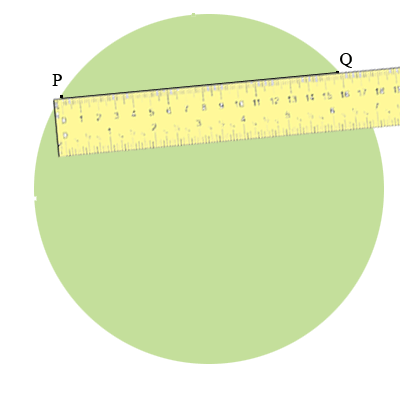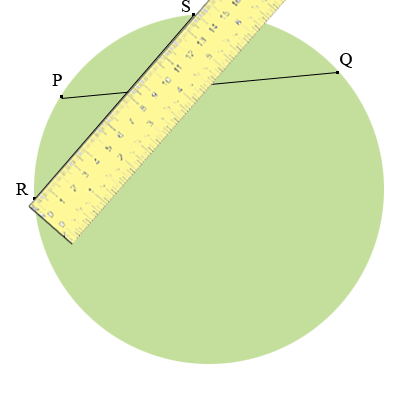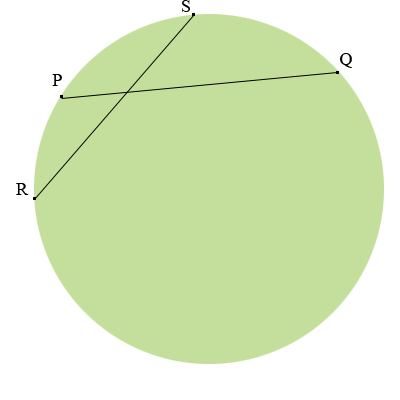SEARCH HOMEMath Central Quandaries & QueriesQuestion from Frank: How to find the length of the radius of a circle if you know the lengths of two chordsHi Frank,

Do you know anything about the chords? From what you sent there is not enough information to determine the radius.
Penny

Thank you for your response. I wondered if maybe there was some formula. What would be the radius of the circle if the lengths of the two chords were 14 cm and 16 cm and the two chords are not perpendicular? Looking forward to hearing from you and once again thank you very much. Frank

Draw a circle with a diameter of more than 16 cm. Mark a point $P$ on the circumference of the circle. Using a ruler find a point $Q$ on the circumference of the circle that is 16 cm from $P.$ Join $P$ and $Q.$Mark a new point $R$ on the circumference of the circle and using a ruler find a point $S$ on the circumference of the circle that is 14 cm from $R.$ Join $R$ and $S.$You now have a chord of length 16 cm and another of length 14 cm.You can do this on any circle with a diameter of more than 16 cm (radius of 8 cm). Thus the fact that there is a chord of length 16 cm and a chord of length 14 cm only tells you that the radius is more than 8 cm.

I hope this helps,
PennyMath Central is supported by the University of Regina and The Pacific Institute for the Mathematical Sciences.